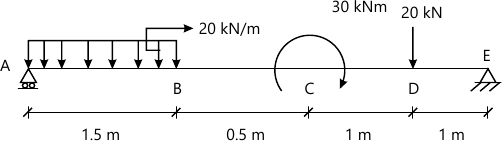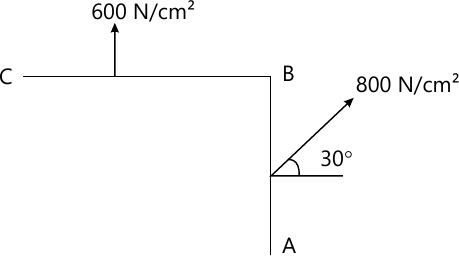MORE IN Strength of Materials
MU Civil Engineering (Semester 3)
Strength of Materials
December 2014
Total marks: --
Total time: --
INSTRUCTIONS
(1) Assume appropriate data and state your reasons
(2) Marks are given to the right of every question
(3) Draw neat diagrams wherever necessary

1 (a) Write the assumptions made in the theory of simple bending.
4 M
1 (b) Establish the relationship between shear force, bending moment and rate-of Loading.
4 M
1 (c) Write a note on Mohr's Circle of stresses.
4 M
1 (d) Establish relationship between young modulus of elasticity, modulus of rigidity and bulk modulus.
4 M
1 (e) A cylindrical shell is subjected to an internal fluid pressure, find an expression E for change in diameter and change in length of cylinder.
4 M

2 (a) Draw a shear force diagram and bending moment diagram for a beam as shown in figures below10 M
2 (b) Explain concept of contra flexture in bending and procedure to locate it.
5 M
2 (c) Explain how to determine the young modulus of elasticity for mild steel and tor steel.
5 M

3 (a) A steel rod of 3cm diameter and 5m long is connected to two grips and the rod is maintained at a temperature of 95°C. Determine the stress and pull exerted when the temperature falls to 30°C, if;
i) The ends do not yield, and
ii) The ends yield by 0.12cm , take E=2×105 MN/mm2 and α=12×10-6/°C.
8 M
3 (b) Distinguish between:
(1) Stress and strain
(2) Force and stress, and
(3) Tensile stress and compressive stress.
6 M
3 (c) Explain with Mehr's Circle method, the procedure for determining the magnitude and direction of shear stress and normal stress on a inclined plane in a member subjected to axial stress of a in horizontal direction: Also find maximum shear stress and its location.
6 M

4 (a) For the state of stress at a point as shown in figure, determine:
(i) The resultant stress on plane BC
(ii) The principal stresses and their directions.
(iii) The maximum shear stresses and their planes.10 M
4 (b) Calculate the instantaneous stress produced in a bar of 10cm2: in area and 3 m long by suddenly application of tensile load of unknown magnitude, if the extension of bar due to suddenly applied load is1.5mm , also determine the suddenly applied load. Take E=2× 105N/mm2
6 M
4 (c) Define resilience, prom resilience and modulus of resilience.
4 M

5 (a) A solid round bar 4m long and 5cm in diameter was found extended 4.6mm under a tensile load of 50kN. This bar is used as a strut with both ends hinged. Determine the buckling load for bar and also the safe load taking factor of safety as 4.
10 M
5 (b) A masonry pier of 3m×4m supports a vertical load of 80kN at an eccentricity of 0.5m along y axis and 1m along x axis respectively
i) Find the stresses developed at each corner of the pier.
ii) what additional load should be placed at the centre of the pier, so that there is no tension anywhere in the pier section>
iii) What are the stresses at the corners with the additional load in the centre?
10 M

6 (a) A cast iron beam with dimensions as given here [ TOP FLANGE: 80×20mm. WEB :20mm×200mm and BOTTOM FLANGE: 160mm ×40mm] The beam is simply supported over a span of 5 meters. If the tensile stress is not to exceed 20N/mm2, find the safe udl which the beam can carry. Find also the maximum compressive stress.
10 M
6 (b) For a T section with dimension given here [ TOP FLANGE: 100×20mm. WEB :20mm×80mm ] Draw shear stress diagram if it is subjected to a shear force of 50kN.
5 M
6 (c) Find the maximum shear stress in a solid circular shaft of a diameter 15cm when transmits 150kW power at 780 r.p.m.
5 M

More question papers from Strength of Materials